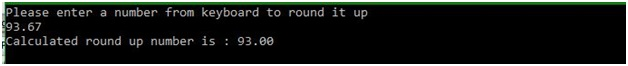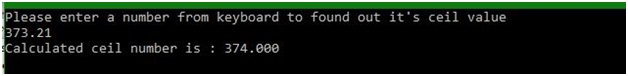# floor() and ceil() functions of math.h in C

In this article, we are going to learn about the floor() and ceil() functions of math.h header file in C language and use them with help of their examples.
Submitted by Manu Jemini, on March 17, 2018

Some basic mathematical calculations are based on the concept of floor and ceiling. Floor means a whole number which should be less than or equal to the number given and must be nearest to the number.

Ceiling means a whole number which is more than or equal to the value given and also must be nearest to the number. You can look at the example given below for more clarification.

To use floor and ceil functions all you need to do is pass a number as a parameter and these function will return a number satisfying the above-explained concept. You can store the result and use it in whichever way you want to.

## math.h - floor() function Example in C

﻿

```#include <stdio.h>
//to use 'floor()' function
#include <math.h>

int main()
{
// set the type of variable
float number, a;

// message for user
printf("Please enter a number from keyboard to round it up\n");
scanf("%f", &number);

// storing the round up value
a = floor(number);

// printing the calculated value
printf("Calculated round up number is : %.2f\n", a);

return 0;
}
```

Output## math.h - ceil() function Example in C

﻿

```#include <stdio.h>
//to use 'ceil()' function
#include< math.h>

int main()
{
// set the type of variable
float number, a;

// message for user
printf("Please enter a number from keyboard to found out it's ceil value\n");
scanf("%f", &number);

// storing the ceil value
a = ceil(number);

// printing the calculated value
printf("Calculated ceil number is : %.3f\n", a);

return 0;
}
```

Output